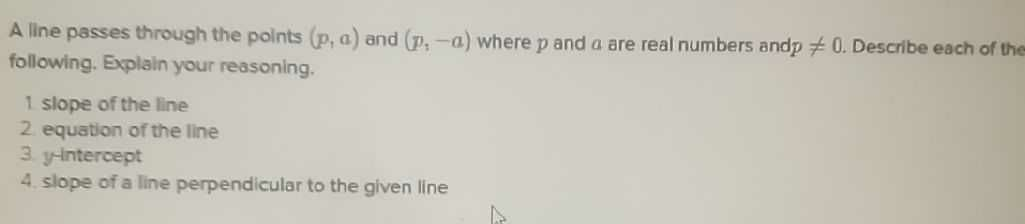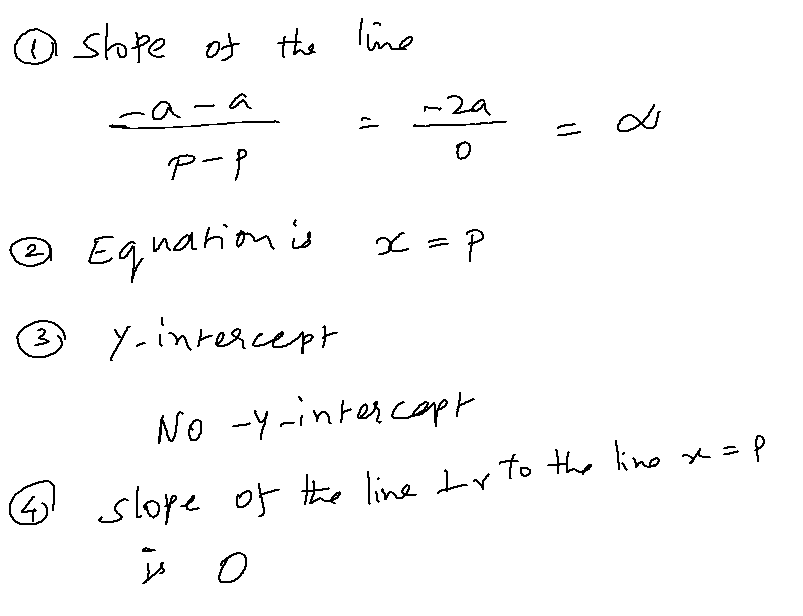### ¿Todavía tienes preguntas de matemáticas?

Pregunte a nuestros tutores expertos
Algebra
PreguntaA line passes through the points $$( p , a )$$ and $$( p , - a )$$ where $$p$$ and $$a$$ are real numbers and $$p \neq 0 .$$ Describe each of the following. Explain your reasoning.

$$1$$ slope of the line 2. equation of the line 3. yntntercept 4. slope of a line perpendicular to the given line